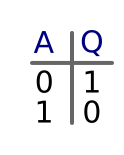# NOT gates

Martin McBride, 2017-01-05
Tags not gate truth table
Categories logic logic gates

An NOT gate is a little different, as it only has one input and one output.

The input can have a value of 0 or 1.

The state of the input controls the state of the output. The output value is 1 if the input is 0, and it is 0 if the input is 1. In other words, the output value is the opposite of the input value.

We call it a NOT gate, because if the input is true, the output is NOT true, and vice versa. NOT gates are sometimes called inverting gates (because they invert the logical sense of the input), and we sometimes use the term negative logic when we are talking about NOT gates. All these different terms mean the same thing.

## Symbol

Here is the symbol for an NOT gate. The input is labelled A, the output is labelled Q.## Truth table

A truth table is a table which shows all possible combinations of input A, and the resulting value of the output, Q. Since there is only one input, there are only 2 possible states:Copyright (c) Axlesoft Ltd 2021